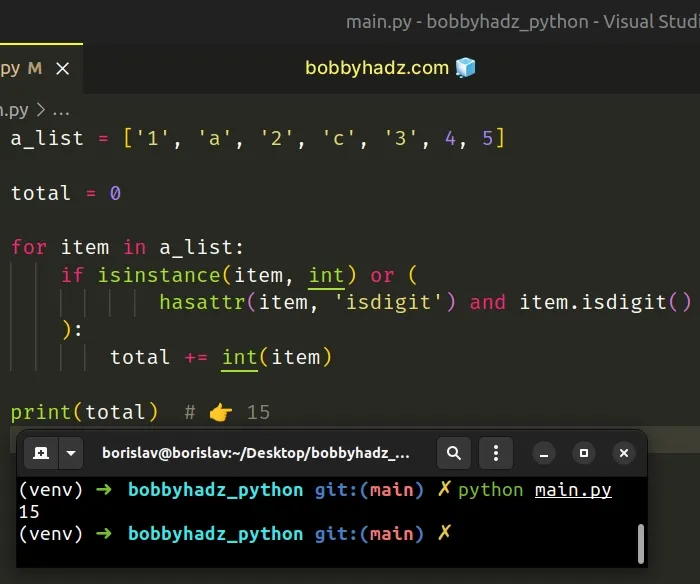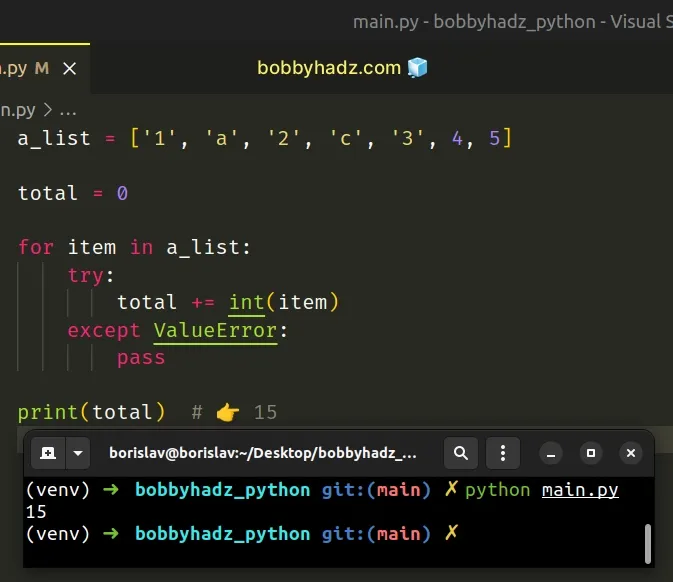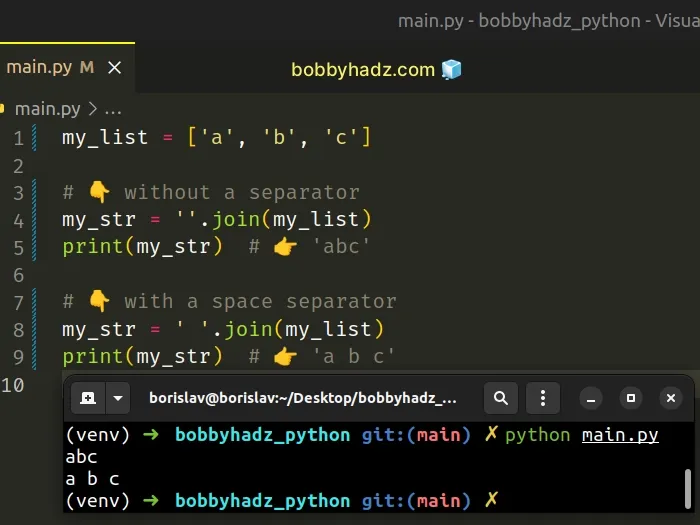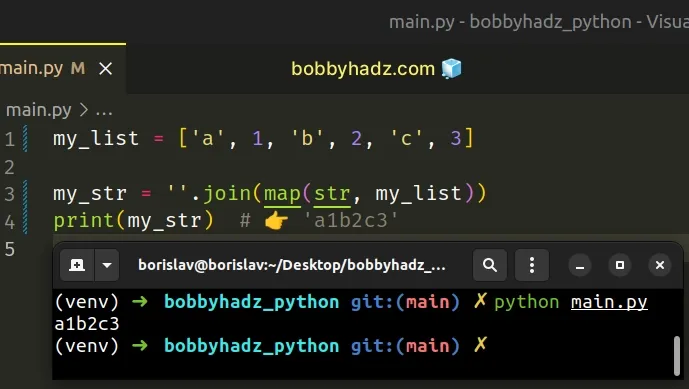# How to sum a List of Strings in PythonLast updated: Feb 19, 2023
5 min## #Sum a List of Strings in Python

To sum a list of strings:

1. Use a `for` loop to iterate over the list.
2. Check if each value is a valid number.
3. Convert the valid numbers to integers or floats and sum them.
main.py
```Copied!```a_list = ['1', 'a', '2', 'c', '3', 4, 5]

total = 0

for item in a_list:
if isinstance(item, int) or (
hasattr(item, 'isdigit') and item.isdigit()
):
total += int(item)

print(total) # 👉️ 15
``````We used a `for` loop to iterate over the list of strings.

On each iteration, we check if the current item is an integer or an integer wrapped in a string.

If the condition is met, we add the value to the `total` variable.

## #Sum a List of Strings using `try/except`

You can also use a try/except statement to sum a list of strings.

main.py
```Copied!```a_list = ['1', 'a', '2', 'c', '3', 4, 5]

total = 0

for item in a_list:
try:
total += int(item)
except ValueError:
pass

print(total) # 👉️ 15
``````On each iteration, we try to convert the current item to an integer.

If a `ValueError` exception is raised, the `except` block runs.

Otherwise, the number gets added to the `total`.

## #Sum a List of Strings using str.join()

This is a three-step process:

1. Call the `str.join()` method on an empty string.
2. Pass the iterable (e.g. a list of strings) to the `join()` method.
3. The result will be a string containing the items of the iterable.
main.py
```Copied!```my_list = ['a', 'b', 'c']

# 👇️ without a separator
my_str = ''.join(my_list)
print(my_str)  # 👉️ 'abc'

# 👇️ with a space separator
my_str = ' '.join(my_list)
print(my_str)  # 👉️ 'a b c'
``````The str.join method takes an iterable as an argument and returns a string which is the concatenation of the strings in the iterable.

Note that the method raises a `TypeError` if there are any non-string values in the iterable.

## #Convert all values to strings before calling join()

If your list contains numbers or other types, convert all of the values to string before calling `join()`.

main.py
```Copied!```my_list = ['a', 1, 'b', 2, 'c', 3]

my_str = ''.join(map(str, my_list))
print(my_str)  # 👉️ 'a1b2c3'
``````The map() function takes a function and an iterable as arguments and calls the function with each item of the iterable.

We used the function to convert each item in the list to a string, before passing the items to the `str.join()` method.

The string the `join()` method is called on is used as the separator between elements.

main.py
```Copied!```my_list = ['a', 'b', 'c']

my_str = '-'.join(my_list)
print(my_str)  # 👉️ 'a-b-c'
``````

If you need to join the list of strings with spaces, call the method on a string that contains a space.

main.py
```Copied!```my_list = ['a', 'b', 'c']

# 👇️ with a space separator
my_str = ' '.join(my_list)
print(my_str)  # 👉️ 'a b c'
``````

If you don't need a separator and just want to join the iterable's elements into a string, call the `join()` method on an empty string.

main.py
```Copied!```my_list = ['a', 'b', 'c']

my_str = ''.join(my_list)
print(my_str)  # 👉️ 'abc'
``````

Alternatively, you can concatenate to a string in a `for` loop.

## #Concatenate to a string in a for loop in Python

This is a three-step process:

1. Declare a variable and initialize it to an empty string.
2. Use a for loop to iterate over the sequence.
3. Reassign the variable to its current value plus the current item.
main.py
```Copied!```my_list = ['bobby', 'hadz', 'com']

my_str = ''

for item in my_list:
my_str += item

``````

The first step is to declare a new variable and initialize it to an empty string.

On each iteration in the `for` loop, we reassign the variable to its current value plus the value of the current list item.

The `+=` operator is a shorthand for `my_str = my_str + item`.

The following code sample achieves the same result.

main.py
```Copied!```my_list = ['bobby', 'hadz', 'com']

my_str = ''

for item in my_list:
my_str = my_str + item

``````

## #Sum the digits in a string in Python

If you need to sum the digits in a string:

1. Use a generator expression to iterate over the string.
2. On each iteration, convert each character to an integer if it is a digit.
3. Use the `sum()` function to get the sum of the digits.
main.py
```Copied!```my_str = '1ab2c3'

# ✅ sum digits in a string that might contain non-digits
total = sum(int(char) for char in my_str if char.isdigit())

print(total)  # 👉️ 6

# -----------------------------------------------------------

# ✅ sum digits in a string that contains only digits

my_str = '246'

total = sum(int(d) for d in my_str)

print(total)  # 👉️ 12
``````

We used a generator expression to iterate over the string.

Generator expressions are used to perform some operation for every element or select a subset of elements that meet a condition.

On each iteration, we check if the character is a digit.

The str.isdigit method returns `True` if all characters in the string are digits and there is at least 1 character, otherwise `False` is returned.

We convert all digits to integers and use the sum() function to get the total.

main.py
```Copied!```my_str = '1ab2c3'

total = sum(int(char) for char in my_str if char.isdigit())

print(total)  # 👉️ 6
``````

The sum function takes an iterable, sums its items from left to right and returns the total.

The `sum` function takes the following 2 arguments:

NameDescription
iterablethe iterable whose items to sum
startsums the `start` value and the items of the iterable. `sum` defaults to `0` (optional)

If your string is guaranteed to only contain digits, you don't have to use the `str.isdigit()` method.

main.py
```Copied!```my_str = '246'

total = sum(int(d) for d in my_str)

print(total)  # 👉️ 12
``````